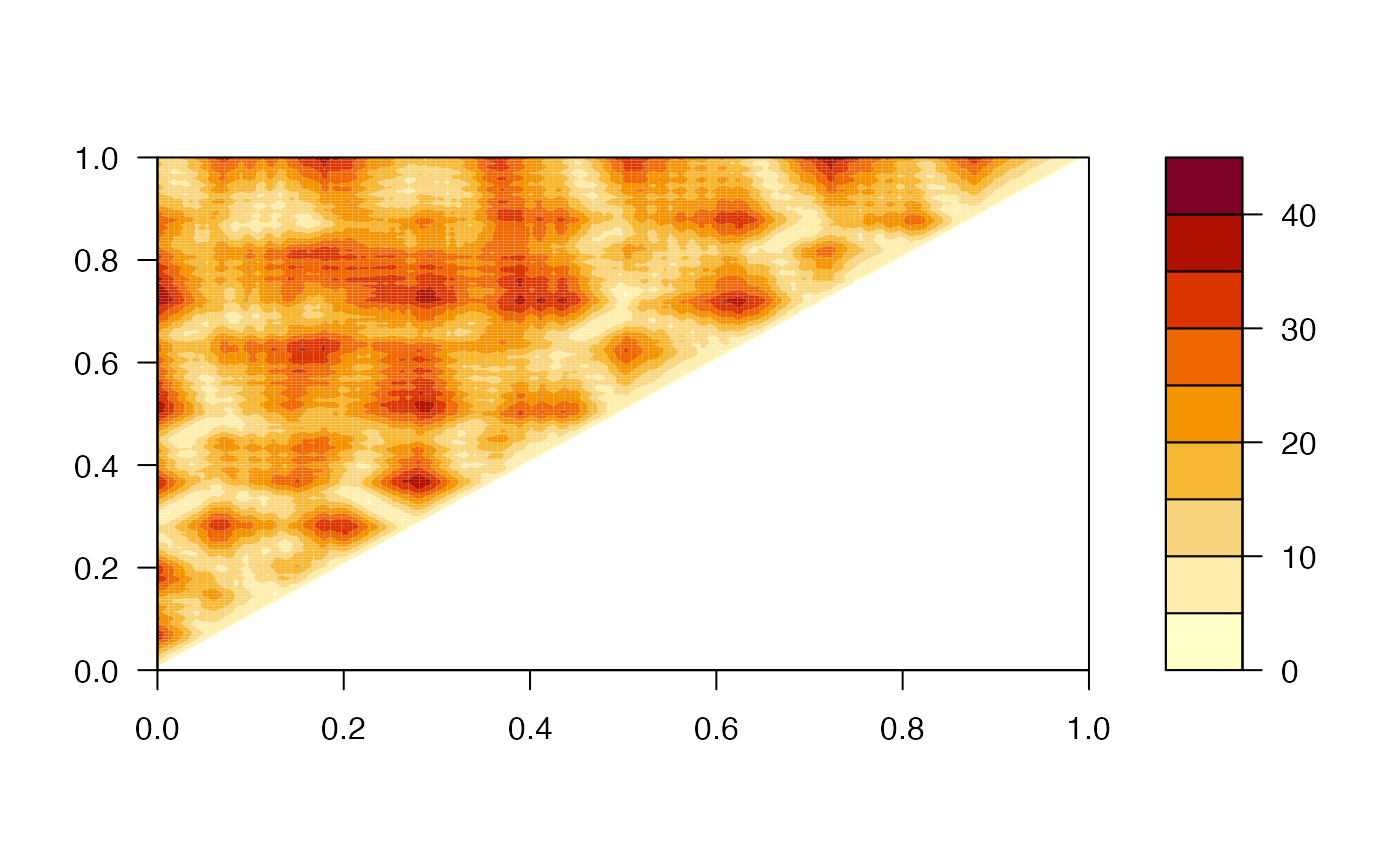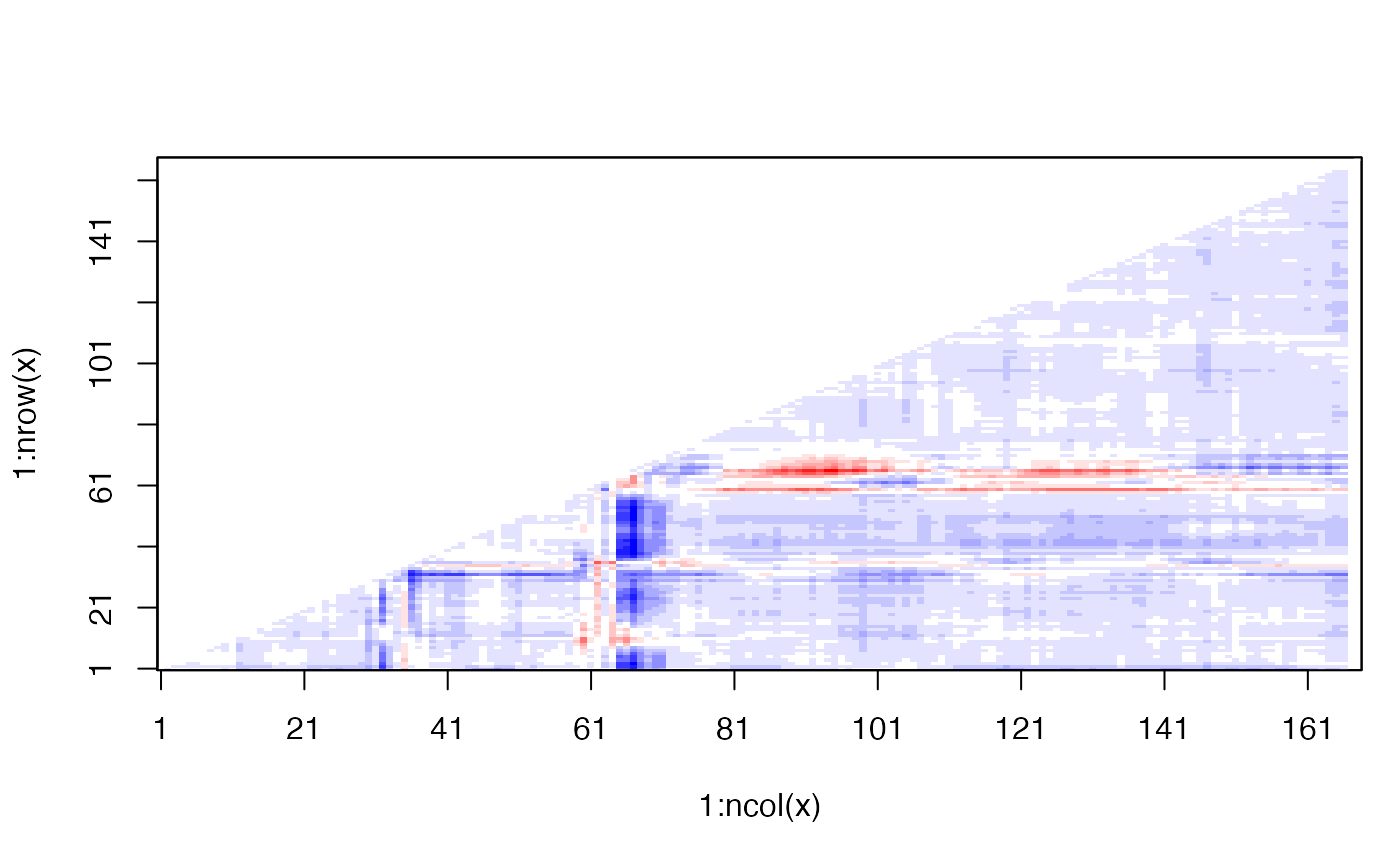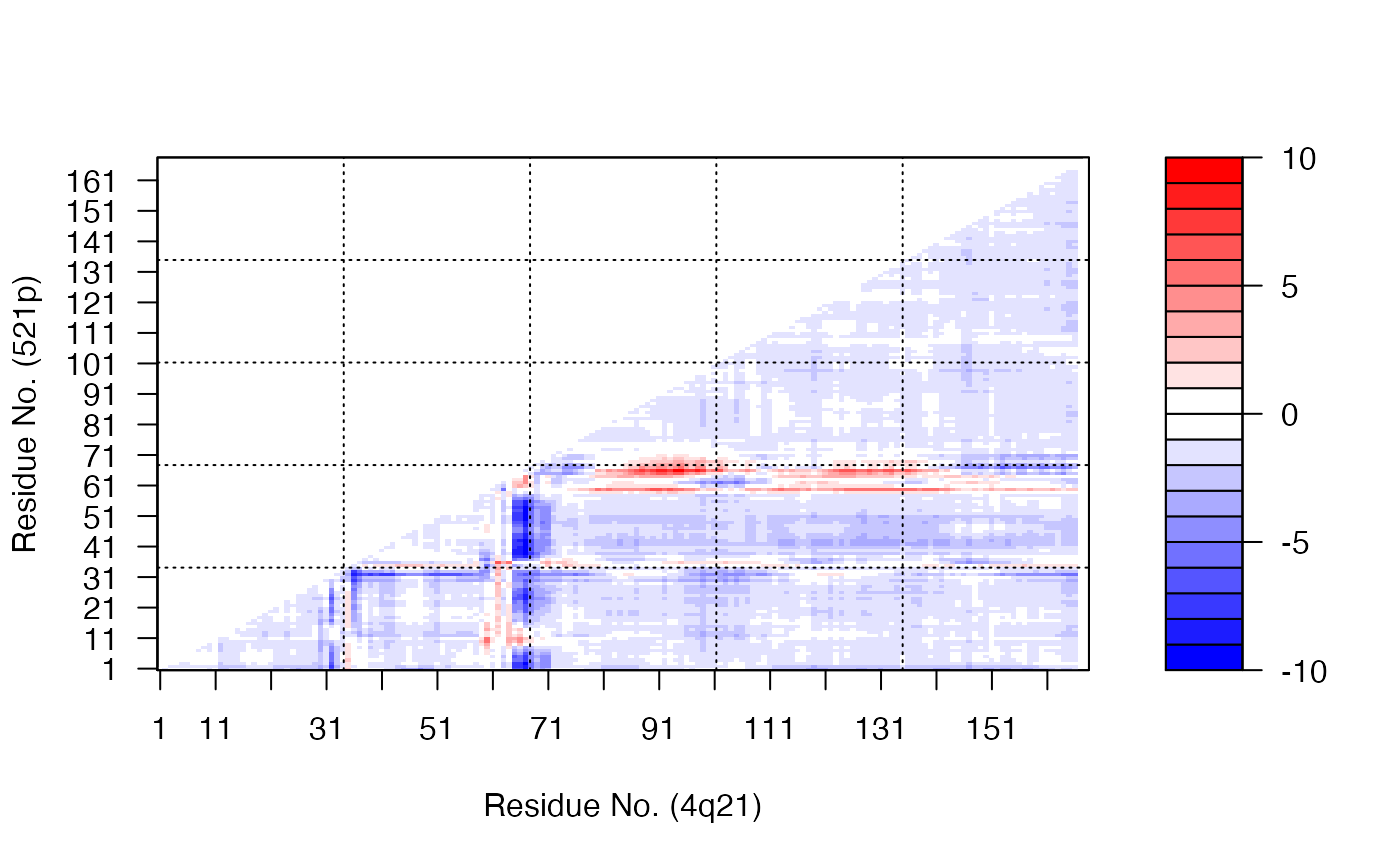Construct a distance matrix for a given protein structure.

dm(...)

# S3 method for pdb
dm(pdb, inds = NULL, grp = TRUE, verbose=TRUE, ...)
# S3 method for pdbs
dm(pdbs, rm.gaps=FALSE, all.atom=FALSE,
aligned.atoms.only=NULL, ...)

# S3 method for xyz
dm(xyz, grpby = NULL, scut = NULL, mask.lower = TRUE,
gc.first=FALSE, ncore=1, ...)

## Arguments

pdb a pdb structure object as returned by read.pdb or a numeric vector of ‘xyz’ coordinates. atom and xyz coordinate indices obtained from atom.select that selects the elements of pdb upon which the calculation should be based. logical, if TRUE atomic distances will be grouped according to their residue membership. See ‘grpby’. logical, if TRUE possible warnings are printed. a ‘pdbs’ object as returned by read.fasta.pdb, read.all, or pdbaln. logical, if TRUE gapped positions are removed in the returned value. logical, if TRUE all-atom coordinates from read.all are used. logical, if TRUE only equivalent (aligned) atoms are considered. Only meaningful when all.atom=TRUE. Default: FALSE. a numeric vector or matrix of Cartesian coordinates. a vector counting connective duplicated elements that indicate the elements of xyz that should be considered as a group (e.g. atoms from a particular residue). a cutoff neighbour value which has the effect of excluding atoms, or groups, that are sequentially within this value. logical, if TRUE the lower matrix elements (i.e. those below the diagonal) are returned as NA. logical, if TRUE will call gc() first before calculation of distance matrix. This is to solve the memory overload problem when ncore > 1 and xyz has many rows/columns, with a bit sacrifice on speed. number of CPU cores used to do the calculation. ncore>1 requires package ‘parallel’ installed. arguments passed to and from functions.

## Details

Distance matrices, also called distance plots or distance maps, are an established means of describing and comparing protein conformations (e.g. Phillips, 1970; Holm, 1993).

A distance matrix is a 2D representation of 3D structure that is independent of the coordinate reference frame and, ignoring chirality, contains enough information to reconstruct the 3D Cartesian coordinates (e.g. Havel, 1983).

## Value

Returns a numeric matrix of class "dmat", with all N by N distances, where N is the number of selected atoms. With multiple frames the output is provided in a three dimensional array.

Grant, B.J. et al. (2006) Bioinformatics 22, 2695--2696.

Phillips (1970) Biochem. Soc. Symp. 31, 11--28.

Holm (1993) J. Mol. Biol. 233, 123--138.

Havel (1983) Bull. Math. Biol. 45, 665--720.

Barry Grant

## Note

The input selection can be any character string or pattern interpretable by the function atom.select. For example, shortcuts "calpha", "back", "all" and selection strings of the form /segment/chain/residue number/residue name/element number/element name/; see atom.select for details.

If a coordinate vector is provided as input (rather than a pdb object) the selection option is redundant and the input vector should be pruned instead to include only desired positions.

plot.dmat, read.pdb, atom.select

## Examples

# \donttest{
# PDB server connection required - testing excluded

##--- Distance Matrix Plot
#>   Note: Accessing on-line PDB file#> Warning: /var/folders/xf/qznxnpf91vb1wm4xwgnbt0xr0000gn/T//Rtmp9oBdbc/4q21.pdb  exists. Skipping downloadk <- dm(pdb,inds="calpha")
filled.contour(k, nlevels = 10)## NOTE: FOLLOWING EXAMPLE NEEDS MUSCLE INSTALLED
if(check.utility("muscle")) {

##--- DDM: Difference Distance Matrix
# Downlaod and align two PDB files
pdbs <- pdbaln( get.pdb( c( "4q21", "521p"), path = tempdir() ), outfile = tempfile() )

# Get distance matrix
a <- dm.xyz(pdbs$xyz[1,]) b <- dm.xyz(pdbs$xyz[2,])

# Calculate DDM
c <- a - b

# Plot DDM
plot(c,key=FALSE, grid=FALSE)

plot(c, axis.tick.space=10,
resnum.1=pdbs$resno[1,], resnum.2=pdbs$resno[2,],
grid.col="black",
xlab="Residue No. (4q21)", ylab="Residue No. (521p)")
}
#> /var/folders/xf/qznxnpf91vb1wm4xwgnbt0xr0000gn/T//Rtmp9oBdbc/4q21.pdb
#> /var/folders/xf/qznxnpf91vb1wm4xwgnbt0xr0000gn/T//Rtmp9oBdbc/521p.pdb
#> ..
#>
#> Extracting sequences
#>
#> pdb/seq: 1   name: /var/folders/xf/qznxnpf91vb1wm4xwgnbt0xr0000gn/T//Rtmp9oBdbc/4q21.pdb
#> pdb/seq: 2   name: /var/folders/xf/qznxnpf91vb1wm4xwgnbt0xr0000gn/T//Rtmp9oBdbc/521p.pdb# }

if (FALSE) {
##-- Residue-wise distance matrix based on the
##   minimal distance between all available atoms
l <- dm.xyz(pdb$xyz, grpby=pdb$atom[,"resno"], scut=3)
}